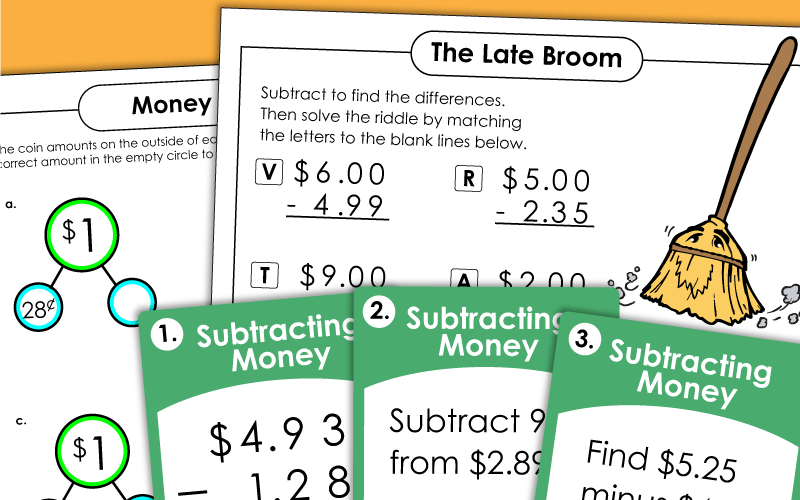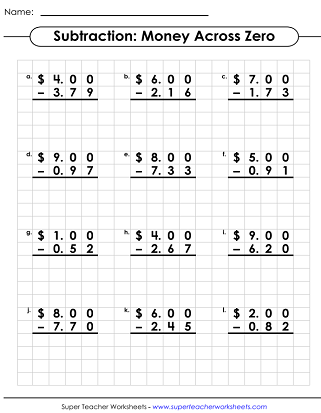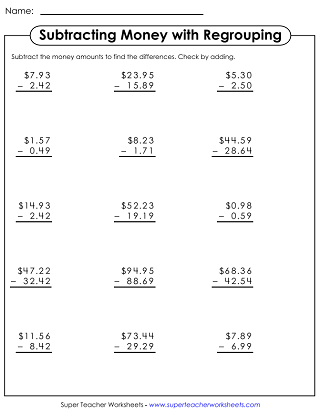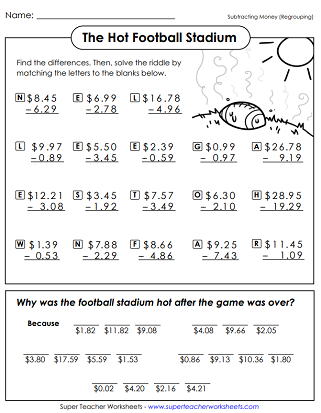# Subtracting Money

Use these worksheets and printable activities to teach students about subtracting money amounts. On this page, you'll find worksheets, task cards, math riddles, and a mini-book.## Subtracting MoneyWorksheets

Why was the football stadium very hot after the game? To discover the answer to this funny riddle, students must subtract pairs of money amounts.
Solve the money subtraction problems to find the funny answer to the riddle: Why was the broom late? These problems require students to subtract across zero. (example: \$5.00-\$3.23)
Subtract what each person buys from the amount they give the cashier.  Requires subtraction across zero.
At the top of the page, students are presented with a series of shapes. They subtract numbers from pairs of shapes and find the differences. For example: Subtract the number in the rhombus from the number in the trapezoid.
Subtract money amounts to find change.  (example: \$5.00 - 4.79)
Multiple-step problems require students to add up the cost of products, then subtract to find change.
Michael receives \$9.99 for his 9th birthday.  Subtract each time Michael spends money to show how much is left.
On this worksheet, students will look carefully at the completed subtraction problems for mistakes. They will explain the mistakes made and solve the problems correctly.
Here's another "Find the Mistakes" worksheet. This one has different problems than the worksheet above.
Subtract the money amounts; Requires regrouping, but does not subtract across zero
Subtraction problems with money amounts; Winter snowmen pictures
Subtracting money on grid paper.  One digit per box ensures kids line up numbers correctly.  (example: \$9.26 - 2.88)
Subtracting money with zero on graph paper.  One digit per box will help kids line up columns.  (example: \$9.00 - 3.48)
Subtract the money amounts on this page.  Includes word problems and standard problems.
Use subtractions to find the missing amount for each number bond. Each bond adds up to \$1, so this skill will require subtracting across zero.
Subtract find the missing amount for each number bond. This is an alternate version of the worksheet above.

## Subtraction WorksheetGenerator

Subtraction Generator

Create your own customized subtraction worksheets with this generator tool. Simply choose the number of digits you'd like in your problems, then write your own header, and the computer will create your own worksheets with random numbers.

4-Digit Subtraction

Practice subtracting 4-digit numbers with these printable worksheets and activities.

More Subtraction

From this page, you can navigate through our huge collection of subtraction worksheets. Includes 2-digit, 3-digit, and 4-digit subtraction. Also includes basic facts as well as fraction and decimal subtraction.

Math Worksheets

Here you can browse though thousands of math worksheets. Topics include addition, multiplication, division, area, perimeter, shapes, symmetry, graphing, money, time, and algebra.

## Sample Worksheet ImagesMy Account
Site Information